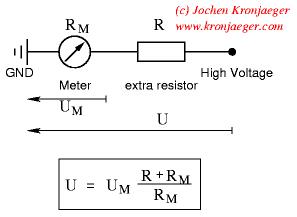## Wednesday, December 29, 2010

### High Voltage Meter or Probe Design

Common voltmeters, digital or analog, usually range to some hundred volts maximum. Higher voltages not only cannot be indicated, but will also destroy the instrument. However, the range of any voltmeter can easily be extended using extra series resistance, as shown in the figure. Calculating the necessary resistance implies knowledge of the input impedance of the voltmeter.Circuit and formula for constructing high voltage probes. For example, let's assume we want to extend the range of a standard digital voltmeter (input impedance 10MOhm) to 100kV. The maximum DC voltage the meter can take is 1000V. This means we need an external 1GOhm high voltage resistor in series with the meter. The total voltage ios given by the value indicatedby the meter, times 100. If we wanted to read the voltage in kV directly, we would need a resistor 1000 times as large as the input impedance of the voltmeter, i.e. 10GOhm.

Such home-brew high voltage probes are good for DC only. For AC voltages, capacitive input impedance of the meter and capacity of the probe must be matched, which is difficult to achieve because of parasitic capacitance of the resistor chain. A few pF (the capacitance of a 1cm radius metallic sphere) make a big difference, especially at higher frequencies.

Source: kronjaeger.com

Skema Rangkaian Elektronika